omG8HI9LSKFW6lhCj8prTs0Z6lUhdOT9Jhi1Sf4m

# How to Easily Find Equivalent Impedance for AC Circuits

The likewise equivalent resistance of dc circuit, we also need equivalent impedance for ac circuit. From its name, equivalent impedance means a single impedance of multiple elements without affecting other elements such as voltage and current source. Equivalent impedance is essential for analyzing the ac circuit.

Make sure to read what is ac circuit first.

## Equivalent Impedance for AC Circuits

Consider the N series-connected impedance shown in Figure.(1). The same current I flows through the impedances. Applying KVL around the loop gives(1)Figure 1. N impedances in series
The equivalent impedance at the input terminals is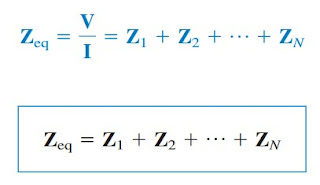(2)
showing that the total or equivalent impedance of series-connected impedances is the sum of the individual impedances. This is similar to the series connection of resistances.

If N = 2, as shown in Figure.(2), the current through the impedances is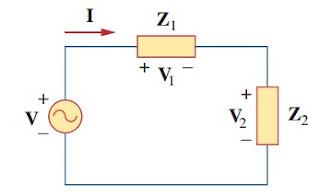Figure 2. Voltage division(3)
Since V1 = Z1I and V2 = Z2I, then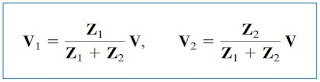(4)
which is the voltage-division relationship.
In the same manner, we can get the equivalent impedance and admittance of the N parallel-connected impedances as can be seen in Figure.(3).Figure 3. N impedances in parallel
The voltage across each impedance is the same. Applying KCL at the top node,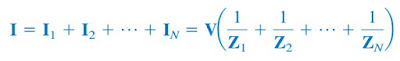(5)
The equivalent impedance is(6)(7)
This indicates that the equivalent admittance of a parallel connection of admittances is the sum of the individual admittances.
When N = 2, as drawn in Figure.(4),Figure 4. Current division
the equivalent impedance becomes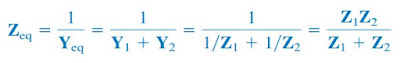(8)
Also, since VZeqI = I1Z1 = I2Z2
the currents in the impedances are(9)
which is the current-division principle.

The delta-to-wye and wye-to-delta transformations that we applied to resistive circuits are also valid for impedances. With the reference to Figure.(5), the conversion formulas are as follows.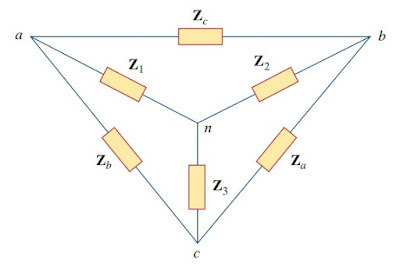Figure 5. Superimposed Y and delta networks
Wye-delta conversion :
A delta or wye circuit is said to be balanced if it has equal impedances in all three branches.
When a delta-wye circuit is balanced, Equations.(10) and (11) become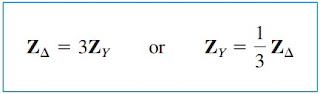(12)
where ZY = Z1 = Z2 = Z3 and ZΔ = Za = Zb = Zc.
As you can see in this post, the principles of voltage division, current division, circuit reduction, impedance equivalence, and wye-delta transformation all apply to ac circuits. In the next post, we will cover another technique for ac circuits such as :
• Superposition
• Nodal analysis
• Mesh analysis
• Source transformation
• Thevenin theorem
• Norton theorem.
The application of sinusoidal circuit and impedance formula results in phase shifter and ac bridge.

## Equivalent Impedance for AC Circuits Examples

For better understanding let us review examples below :
1. Find the input impedance of the circuit in Figure.(6). Assume that the circuit operates at ω = 50 rad/s.
Let
Z1 = Impedance of the 2 mF capacitor
Z2 = Impedance of the 3 Ω resistor in series with the 10 mF capacitor
Z3 = Impedance of the 0.2 H inductor in series with 8 Ω resistor
Then
2. Determine vo(t) in the circuit of Figure.(7)
To do analysis in the frequency domain, we must first transform the domain circuit in Figure.(7) to the phasor domain equivalent in Figure.(8). The transformation produces
Z1 = Impedance of the 60 Ω resistor
Z2 = Impedance of the parallel combination of the 10 mF capacitor and 5 H inductor.
3. Find current I in the circuit of Figure.(9).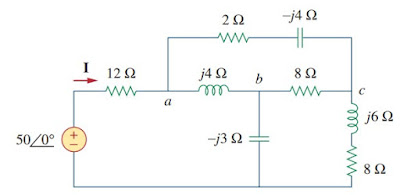Figure 9
Solution :
The delta network connected to nodes a, b, and c can be converted to the Y network of Figure.(10). We obtain the Y impedances as follows using Equation.(11) :
The total impedance at the source terminals is
The desired current isFigure 10

Have you understood what is impedance formula?  Don't forget to share and subscribe! Happy learning!
Reference:  Fundamentals of electric circuits by Charles K. Alexander and Matthew N. O. Sadiku

Untuk Bahasa Indonesia baca Rumus Impedansi Ekuivalen Rangkaian AC
Related Posts
SHARE